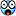## Recommended Posts

I find fringe mathematics fascinating.

There are equations like e^(pi*i) = -1 that boggle my mind and proofs for how 0.999... = 1 that just amaze me.

I thought it would be cool to start a thread for people to post proofs/facts/interesting problems in math that warp our common sense notions about how numbers work.

I'll start with the proof that .999[bar]=1 (where [bar] denotes an infinite series):

x=.999[bar]

10x=9.99[bar]

10x-x=9.99[bar]-.999[bar]

9x=9

x=1

##### Share on other sites

• 1 month later...

Wiles proof that $x^n+y^n=z^n, n>2 --> xyz=0$

Lebiniz and his most elegant equation $pi/4=1-(1/3)+(1/5)-(1/7)....$

##### Share on other sites

As far as calculations for π go, by far the most awesome although completely useless is the Buffon Needle experiment.

##### Share on other sites

• 4 weeks later...
e^(pi*i) = -1

Can you move that around so that "pi =" is on the left?

There is also a way involving iterative sin of a number that gets pi.

##### Share on other sites

x=.999[bar]

10x=9.99[bar]

10x-x=9.99[bar]-.999[bar]

9x=9

x=1

An alternative approach (using fractions):

$\frac13 = 0.33333...$

$\frac13 \times 3 = (0.33333...) \times 3$

$1 = 0.99999...$##### Share on other sites

Hmmm... Not quite. I was just posting an alternative proof. This thread is about (Quoted OP):

I thought it would be cool to start a thread for people to post proofs/facts/interesting problems in math that warp our common sense notions about how numbers work.

## Create an account

Register a new account